International Journal of Intelligent Information Systems
Volume 5, Issue 3-1, May 2016, Pages: 1-4

Applying the Fuzzy Ant-Miner Algorithm to Extract the Success Indicators of Balloon Dilation in PA-IVS

Mohamed Hamlich*, Mohammed Ramdani

Computer science lab, UH2, FSTM, Mohammedia, Morocco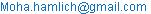(M. Hamlich)

Mohamed Hamlich, Mohammed Ramdani. Applying the Fuzzy Ant-Miner Algorithm to Extract the Success Indicators of Balloon Dilation in PA-IVS. International Journal of Intelligent Information Systems. Special Issue: Smart Applications and Data Analysis for Smart Cities. Vol. 5, No. 3-1, 2016, pp. 1-4. doi: 10.11648/j.ijiis.s.2016050301.11

Received: October 10, 2015; Accepted: October 12, 2015; Published: June 18, 2016

Abstract: Several studies have sought to identify the parameters that determine the outcome of balloon dilation in pulmonary atresia with ventricular septum. However, none of these studies was based on the ant colony algorithm. In this paper we focus on the implementation of an algorithm based on ant colonies: Fuzzy Ant-Miner. This method uses the concepts of fuzzy logic to extract rules from the training data. These rules are exploited using a Mamdani fuzzy inference system for classification and prediction. The results obtained by this method in the form of fuzzy rules are easy to interpret, and close to human reasoning.

Keywords: Atresia with Intact Ventricular Septum, Balloon Dilation, Fuzzy Ant-Miner, Fuzzy Partitions, Fuzzy Rules

Contents

1. Introduction

The decision tree algorithms present the disadvantage of using sequential variables (non-simultaneous). On the other hand, the passage from a tree node to another, divides the attribute into two separate partitions in a sudden manner: noise superimposed on the discretization value can generate errors.

To overcome these drawbacks, we used a method based on ant colonies algorithms: Fuzzy Ant- Miner . This is an extension of the cant- Miner  algorithm that extracts fuzzy rules from training data. It can handle both nominal and continuous data, which is an interesting improvement compared to other methods based on ant colonies like Ant- Miner .

The rest of the paper is organized in three sections:

The first is devoted to the explanation of the Fuzzy Ant- Miner method. The subsequent analysis will focus on the results achieved by decision trees and those obtained by the Fuzzy Ant- Miner method. In the third section, we analyze the classification of new instances using a fuzzy inference system.

2. Fuzzy Ant-Miner

A.  Fuzzy discretization

In order to avoid the crisp discretization used by cAnt-Miner, our method discretizes the values of a continous attribute into fuzzy partitions . First a threshold x is determined in the same way as cAnt-Miner (the one that leads to the minimum entropy). We assume that the threshold is a fuzzy number around x. Then the values of the continuous attribute are transformed into membership degrees to the fuzzy values:

Ai << x (low), Ai ≈ x (average) and Ai >> x (high).

The membership function of the continuous attribute Ai to a fuzzy value Ai << x is calculated using Equation (1) with reference to FIG. 1.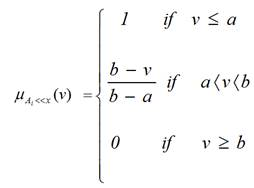(1)

And the membership functions of the continuous attribute Ai to a fuzzy value Ai ≈ x are calculated by the equation (2):(2)

Similarly, the membership functions of the continuous attribute Ai to a fuzzy value Ai>> x are calculated by: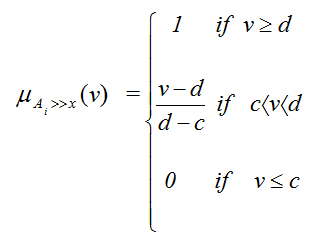(3)

Where parameters (a, b, c and d) determine the boundaries of the fuzzy area and so the degree of fuzziness. These parameters are determined in the same way as the threshold x (value that gives the minimum entropy in a given partition). Note that other ways of generating the fuzzy partitions can be used in our method.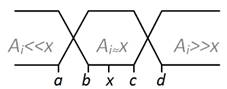Fig. 1. Fuzzy discretization of a continuous attribute.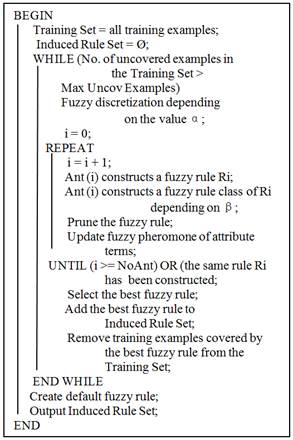Fig. 2. Pseudo-code of Fuzzy Ant-Miner algorithm.

B.  Fuzzy Ant-Miner Algorithm

The In order to deal with fuzzy concepts we have extended the algorithm Ant-miner to treat these concepts. The pseudo code of Fuzzy Ant-Miner method is presented in the Figure 2.

The method begins with all learning examples and an empty list of rules. Fuzzy discretization is performed on the fly  in the outer loop (While). The inner loop (Repeat. .. Until) finds a fuzzy rule, simplifies and updates the pheromone levels in different terms. This rule will be elected (stored in the list of rules) if its quality is the highest, in this case all the examples covered by this rule will be removed from the training set. A sample is removed from the training set if the rule covers this example with a number greater than a certain degree β. The rule extraction process stops when the training set  contains only a number of examples less than a number entered by the user. The number of iterations of the inner loop is equal to the number of ants if there's no convergence (the same rule found several times).

C.  Rule pruning

After obtaining the complete rule, the method proceeds to pruning it . The pruning process is based on the quality of the fuzzy rule. Indeed the quality is computed with TP* (fuzzy true positive), FP*(fuzzy false positive), FN* (fuzzy false negative) and TN* (fuzzy true negative) that are determined by calculating the membership degree of each term in the rule.

The eth example Oe of the training set is defined by: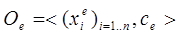Where:

(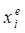)i=1….n represents the attribute values of the example.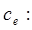Class of the example.

Each rule rm obtained by our method is defined by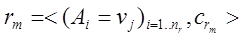Where:

vj is the fuzzy value

nr is the number of terms in the rule.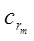: Class predicted by the best rule obtained.

To determine the quality of the fuzzy rule, the examples are injected into the rule:

The filter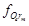of the example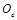in the rule is defined by the membership degree of a term xieto a fuzzy value vj and the membership degree of a class example to a class rule: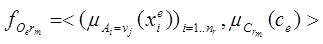A fuzzy true positive value of exampleis calculated by: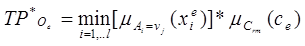(4)

A TP* value of a training set is: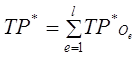(5)

Similarly we calculate FP*, TN* and FN*: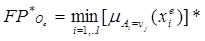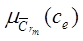(6)

Where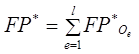(7)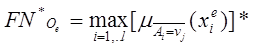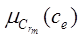(8)

Where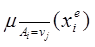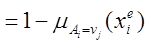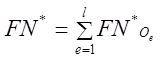(9)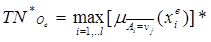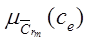(10)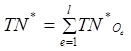(11)

The fuzzy quality Q* is then calculated by: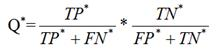(12)

Before pruning the rule, the class is determined by the most frequent class among the examples covered by the rule in the training set: The rule covers the example Oe if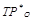Eq. 4 is higher than a parameter β. This parameter (between 0 and 1) is adjusted for better performance. It filters the examples covered by the rule. If β = 0 then all examples of which an attribute has a value within the fuzzy area are covered by the rule. If β = 1 then all examples of which an attribute has a value within the fuzzy area are not covered by the rule. The user sets this parameter for the best accuracy. In each rule pruning iteration, every term is temporarily removed from the rule (note that a change in a rule antecedent may result in a change of the rule consequent), and the quality of the rule is re-evaluated. At the end of the iteration, only the term removal of which leads to an improvement of the rule quality is actually removed. The process of rule pruning stops when there is only one term left, or each term removal no longer leads to an improvement of rule quality. Once the rule pruning has been performed, the artificial ant increases the level of pheromone of the terms still present in the rule antecedent according to the fuzzy quality of the rule: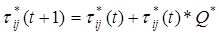(13)

The results obtained with Fuzzy Ant-Miner showed an obvious improvement compared to those of Ant-Miner and cAnt-Miner.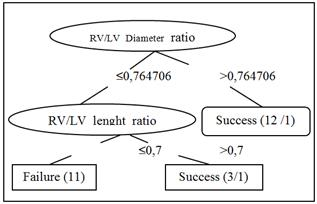Fig. 3. Decision tree generated by J48 algorithm using the Weka software.

D.  Results

This data set is composed of 26 echocardiograms  done on patients diagnosed with PA-IVS who underwent balloon dilatation. Each instance consists of 32 numeric attributes, two nominal attributes and a nominal class that indicates the success or failure of the intervention. The small number of cases of all the data that was provided to us, is the consequence of the fact that congenital heart disease is rare.

The tree generated by the J48 algorithm  is shown in Figure 3. This tree has the disadvantage of using sequential variables (non-simultaneous: we must first test attribute:   RV / LV_diameter_ratio), and if a variable changes in the tree, then all the tree changes. On the other hand, the passage from a tree node to another, divides the attribute into two separate partitions in a sudden manner: noise superimposed on the value of discretization can generate errors (eg partitions: RV / LV_diameter_ratio> 0.764706 and RV / LV_diameter_ratio ≤ 0.764706).

Results of Fuzzy Ant-Miner method

The Fuzzy Ant-Miner algorithm uses a fuzzy discretization of continuous attributes and extract rules that will be exploited by using the fuzzy inference systems for classification and prediction . The results obtained by this method in the form of fuzzy rules are easy to interpret, and close to human reasoning. The average predictive accuracy obtained is 0.7862. The results obtained by this method as three fuzzy rules are noted below:

IF ratiolen is Low (a=0.620500 || b=0.755176) && IF ratiodia is Low (a=0.652807 || b=0.861898), the result is: failure

IF ratiodia is High (a=0.700000 || b=0.900000), the result is: success

IF ratiolen is High (a=0.710024 || b=0.761486) && IF ratiotm is Low (a=0.825595 || b=0.882738), the result is: success

The fuzzy rules 1 and 2 justify the tree generated by the J48 algorithm, while overcoming the disadvantages of decision trees already mentioned. Our approach has generated rule 3 which uses a new term (ratiotm). The attributes contained in the rules are: ratiodiam, ratiolen and ratiotm. The learning examples involving these attributes and their coverage by the rules are given in Table 1.

Table 1. Class’S predictions of six examples by the three fuzzy rules obtained.

 Sample Ratio lengt Ratio diam Ratio tm Classs Rule 1 Rule 2 Rule 3 Class predicted 1 0.8 0.94 0.9 s 0 1 0 1*s f 0 0 0 2 0.58 0.62 0.808 s 0 0 0 1*f f 1 0 0 3 0.864 0.68 0.75 s 0 0 1 1*s f 0 0 0 4 0.62 0.8 0.722 s 0 0.5 0 0,5*s f 0,3 0 0 5 0.72 0.64 0.70 s 0 0 0. 2 0,26*f f 0.26 0 0 6 0.72 O,8 0,8 s 0 0.5 0.2 0,5*s f 0,26 0 0

s:success, f:failure

3. Fuzzy Inference

The fuzzy rules generalized by Fuzzy Ant-Miner algorithm from the training set are used to classify new examples . This classification is based on the principle of fuzzy inference system. Indeed each rule obtained, that involves a fuzzy term from the continuous attribute, has a degree of validity . This degree depends on the fuzzy probabilities. An example filtered by this rule will have the class of this rule with a degree of uncertainty calculated using the principle of generalized modus ponens. The fuzzy inference system used is the SIF Mamdani.

The output class is constructed by aggregating the classes obtained by each rule. We deem that the rules are linked by a logical "OR", and therefore we calculate the maximum of the resulting membership functions for each rule. Since the output is categorical, we affect the class of the rule, with the highest output value, to the injected example.

Table 1 presents six examples injected into our method and whose class is predicted with a degree of uncertainty.

In our case, Example 6 will be assigned to the class of rule 2 (success) with a level of 50%: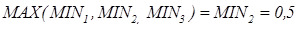4. Conclusion

Fuzzy Ant-Miner is an extension to the learning algorithms based on ant colonies in order to process continuous valued attributes. These parameters are discretized on the fly according to the concepts of fuzzy logic.

We got three fuzzy rules by applying the algorithm Fuzzy Ant-Miner on these medical data.

The domain expert has confirmed that the rules obtained by Fuzzy Ant-Miner reflect their way of thinking in the face of confusing situations: that is to say, when the attribute value is exactly equal to the decision threshold.

References

1. A.Drighil, M.Aljufan, A.Slimi, S.Yamani, J.Mathewson, F.AlFadly", Echocardiographic determinants of successful balloon dilation in pulmonary atresia with intact ventricular septum", European Journal of Echocardiography (2010) 11, 172–175 doi:10.1093/ejechocard/jep193
2. Parpinelli, R., Lopes, H., Freitas, A. (2002)."Data mining with an ant colony optimization algorithm," IEEE. Transactions on Evolutionary Computation, vol. 6, no. 4, pp. 321–332.
3. M.Hamlichand M.Ramdani, "Data classification by Fuzzy Ant-Miner", IJCSI International Journal of Computer Sciences issues, Vol 9, Issue 2, N° 3, Marsh 2012, ISSN (Online) 1694-08 14..
4. M.Hamlich and, M.Ramdani, "Scout Ants for Clustering", Journal of Theoretical and Applied Information Technology (JATIT), September 2013 -- Vol. 55. No. 1 -- 2013.
5. M.Hamlich and, M.Ramdani, "Ant Colony algorithms for data learning", International Journal of Applied Evolutionary Computation (IJAEC), Volume 4, Issue 3, pp 1-10. July-September 2013.
6. M.Hamlich and M.Ramdani, «Improved ant colony algorithms for data classification», ISBN: 978-1-4673-4764-8, IEEE Xplore.
7. M.Hamlich and, M.Ramdani, "Fuzzy classification by ant colonies", Seventh Iinternational Conference on Intelligent Systems: Theories and Applications (SITA'12) 16-17 MAY 2012, Mohammedia, Morocco.
8. M.Hamlich and, M.Ramdani, "Fuzzy Ant-Miner", IADIS European Conference Data Mining 2012,Lisboa Portugal, ISBN 978-972-8939-69-4.
9. Otero,F. Freitas,A, and C.G.Johnson (2008), "cAnt-Miner: an ant colony classification algorithm to cope with continuous attributes," in Ant Colony Optimization and Swarm Intelligence, LNCS 5217. Springer, pp. 48–59.
10. M.Hamlich and M.Ramdani, «Improved ant colony algorithms for data classification », International Conference on Complex Systems (ICCS), Agadir, Morocco, ISBN: 978-1-4673-4764-8, November 05-06, 2012.

 Contents 1. 2. 3. 4.
Article ToolsAbstractPDF(528K)